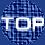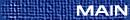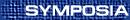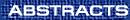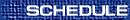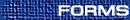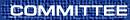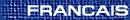Analysis / Analyse
(T. Ransford, Organizer)

L. BARIBEAU, Université Laval, Québec  G1K 7P4
Iterated function systems and the l-lemma

To any finite family of contractions fi: z®ci(l)z+bi(l) can be associated its so-called attractor, which is the unique non-empty compact subset of the z-plane which is invariant under all the fi's. For instance, for the pair of maps z® z/3 and z® z/3+2/3, the attractor is the celebrated Cantor set. We will talk about the analytic properties of the attractor when the ci's and bi's are holomorphic, and discuss this in the context of the l-lemma of Mañé, Sad and Sullivan.

ALAIN BOURGET, Johns Hopkins, 3400 North Charles Street, Baltimore, Maryland  21218, USA
Nodal statistics for the Lamé ensemble

Recently, there has been great interest in both mathematics and physics circles in the asymptotic statistics of both eigenvalues and zeroes of eigenfunctions of quantum Hamiltonian. Despite the abundance of conjectures, we present some rigorous results concerning the asymptotic statistics of the zeroes of the joint eigenfunctions (Lameé harmonics) of an important class of quantum completely integrable dynamical system: the Gaudin spin chains with real marked points.

A. BRUDNYI, University of Calgary, Calgary, Alberta  T2N 1N4
Bounded analytic functions on special Riemann surfaces

Let M be a non-compact connected Riemann surface of finite type, and R Ì Ì M be a relatively compact domain such that H1(M,Z) = H1(R,Z). Let [(R)\tilde]® R be a covering and i: U\hookrightarrow[(R)\tilde] be a subdomain such that the induced homomorphism i* of fundamental groups is injective. We study the algebra H¥(U) of bounded holomorphic functions on U. We will show that many properties of H¥(U) are essentially the same as those of H¥(D), where D Ì C is the open unit disk. In particular, we prove for H¥(U): a Forelli type theorem on projections, some theorems on the characterization of interpolating sequences, an extension theorem for matrices with entries in H¥(U) (= a non-linear version of the corona theorem). Our methods are of a geometric nature and have never been previously used in the theory of H¥.

Y. CHEN, Lakehead University, Thunder Bay, Ontario  P7B 5E1
An improved bound for spectral variation near algebraic elements

Let A be a Banach algebra and a, b two algebraic elements of degree at most 2 of A. Our earlier work showed that the Hausdorff distance between the spectra s(a) and s(b) satisfies the following inequality:

 D2 æè s(a),s(b) öø £ c max (||a||, ||b||)||b-a||,
where c £ 3. In this talk, we will improve the bound by showing that c £ Ö3+1.

B. COLE, Brown University
Polynomial formulas for the disk and the bidisk

We produce polynomial formulas for the disk and the bidisk related to the Pick and Agler interpolation problems. These formulas can be used to deduce the von Neumann inequalities for polynomials in one and two variables applied to linear operators on Hilbert space. This is joint work with John Wermer.

G. DAFNI, Concordia University, Montreal, Quebec  H3G 1M8
A duality theorem for some function spaces related to H1 and BMO

We consider the function spaces Qa, 0 < a < 1, on n dimensional Euclidean space, and identify them with the duals of certain spaces of distributions which contain the Hardy space H1. The proof uses generalized Carleson measures, square functions, and Hausdorff capacity. An atomic decomposition for the predual is also obtained.

R. FOURNIER, Department of Mathematics, Dawson College, Montreal, Quebec  H3Z 1A4
On certain spaces of metrics of hyperbolic type

Let D:={ z | |z| < 1} be the open unit disc of the complex plane, H(D) the set of functions analytic in D endowed with the usual topology and B :={ w Î H(D) | w(D) Í D}. For each w Î B we define w* (z) = |w¢(z)|/(1-|w(z)|2) and W :={ w Î B | supz Î D w*(z) < ¥}. Given an outer function h Î B let Wh : = { w Î W | w*(z) £ h(z), z Î D}. In this talk we shall study a natural homeomorphism T : Wh ® B and elementary properties of the extreme points of Wh.

ANDREA FRASER, Dalhousie University
A characterization of some singular integral operators on the Heisenberg group

The Heisenberg group Hn is the simplest example after Rn of a homogeneous group; that is, a group which admits a family of automorphic dilations. I consider the class of standard Calderón-Zygmund singular integral operators on Hn. In particular, this class includes the Cauchy-Szegö projection. The standard singular integral operators with radial convolution kernels form a sub-class of the Marcinkiewicz-type spectral multipliers, characterized by Müller, Ricci, and Stein in 1995. I establish a stricter condition on the multipliers which characterizes this sub-class.

P. GAUTHIER, Universite de Montreal, CP 6128 Succ. Centre-Ville, Montreal, Quebec  H3C 3J7
The existence of universal inner functions on the unit ball of Cn

Together with XIAO Jie, we have shown that there exists an inner function I on the unit ball Bn of Cn such that each function holomorphic on Bn and bounded by 1 can be approximated by non-Euclidean translates'' of I.

F. LARUSSON, Department of Mathematics, University of Western Ontario, London, Ontario  N6A 5B7
Recent developments in the theory of disc functionals

We describe recent work with Ragnar Sigurdsson in which we show that a disc functional on a complex manifold has a plurisubharmonic envelope if all its pullbacks by holomorphic submersions from domains of holomorphy in affine space do and it is locally bounded above and upper semicontinuous in a certain weak sense. For naturally defined classes of disc functionals on manifolds, this result reduces a property somewhat stronger than having a plurisubharmonic envelope to the affine case. The proof uses a recent Stein neighbourhood construction of Rosay, who proved the plurisubharmonicity of the Poisson envelope on all manifolds. As a consequence, the Riesz envelope and the Lelong envelope are plurisubharmonic on all manifolds; for the former, we make use of new work of Edigarian. The basic theory of the three main classes of disc functionals is thereby extended to all manifolds.

N. LEVENBERG, Auckland University/Syracuse University
Distribution of nodes on algebraic curves in CN

Given an irreducible algebraic curve A in CN, let md be the dimension of the complex vector space of all holomorphic polynomials of degree at most d restricted to A. Let K be a nonpolar compact subset of A, and for each d=1,2,..., choose md points {Adj}j=1,...,md in K. Finally, let Ld be the d-th Lebesgue constant of the array {Adj}; i.e., Ld is the operator norm of the Lagrange interpolation operator Ld acting on C(K), where Ld(f) is the Lagrange interpolating polynomial for f of degree d at the points {Adj}j=1,...,md. Using techniques of pluripotential theory, we show that there is a probability measure mK supported on K such that for any array in K satisfying limsupd® ¥Ld1/d £ 1, the discrete measures

 md:= 1md mdå j=1 dAdj,     d=1,2,...,
converge weak-* to mK.

J. MASHREGHI, Universite Laval, Quebec, G1K 7P4
Argument of outer functions

While a complete description of the modulus of an outer function is known, a satisfactory characterization of the argument is not available. Being interested in characterizing real function y such that exp(iy(x)) = O(x)/|O(x)|, for almost all x Î R, and for some outer function O, we show that if y is a sawtooth-Lipschitz function, then it is the argument of an outer function on the real line.

PETR PARAMONOV, Moscow State University, Moscow  119899, Russia
Uniform approximation of (stationary) gravitational fields. Extension and related capacity

Capacitative criteria for the approximation of (stationary) gravitational fields on compact subsets of R3 will be given. Subadditivity and density properties of the related capacity will be established. Extension from balls to all of R3 will also be discussed.

E. POLETSKY, Syracuse University
Analytic multifunctions and Jensen measures

In the talk we will give a characterization of analytic multifunctions through Jensen measures and discuss consequences. This approach allows us to use methods of pluripotential theory to study the geometry of multifunctions.

D. ROCHON, Université du Québec à Trois-Rivières, Département de Mathématiques et d'informatique, Trois-Rivières, Québec  G9A 5H7
A Cantor set for bicomplex numbers

This talk will be about a commutative generalization of the complex numbers called bicomplex numbers. First, we will focus our attention on the bicomplex dynamics. In particular, we will give a generalization of the Mandelbrot set and the Julia sets in dimensions three and four and we will establish some relationships between them. Furthermore, from the properties of the Julia sets we will propose a bicomplex Cantor set in dimension four.

J. ROSTAND, Centre de recherches pour la defense, Ottawa, Ontario
Sur les automorphismes de la boule unité spectrale / On the automorphisms of the spectral unit ball

Soit Wn l'ensemble des matrices n×n dont le rayon spectral est inférieur à 1. Le but ultime est de répertorier l'ensemble des automorphismes de Wn. Dans un premier temps, nous discuterons des résultats connus dans le cas général. Puis, nous porterons notre attention sur le cas particulier n=2 et verrons qu'il est possible d'en savoir davantage.

Let Wn be the set of n×n matrices with spectral radius less than 1. The ultimate goal is to make a list of all automorphisms of Wn. We will first present what has been done in the general case. Then we will focus on the special case n=2 and see what more can be obtained.

(Transparencies will be in english et l'exposé en français.)

ANATOLY SEDLETSKII, Moscow State University, Moscow  119899, Russia
Muntz-Szasz type approximation

The problem of the description for complete systems of powers ( with complex exponents) in the Lebesgue spaces on a finite interval will be discussed.

ZBIGNIEW SLODKOWSKI, Department of Mathematics, Statistics and Computer Science, University of Illinois at Chicago, Chicago, Illinois  60607, USA
Evolution of compact subsets of C2 by Levi curvature

(Text of abstract:) The talk will report on the joint work of the speaker with Giuseppe Tomassini(Pisa). Informally speaking, we consider a type of evolution in which a domain bounded by a smooth hypersurface expands at a strictly pseudoconcave point in the direction of the outside normal vector at a rate proportional to the negative of its Levi curvature at the point, but its rate of change is zero at the weakly pseudoconvex points. Formal definition of such evolution is given in terms of weak (viscosity) solutions of a certain nonlinear, degenerate parabolic equation and global existence and uniqueness is obtained for every compact subset K of C2. We show that this evolution is continuous in time, nondecreasing and that the sets added in time to the initial one have local maximum property with respect to plurisubharmonic functions. Thus the limit of the evolution is a kind of a hull; we examine its relation the Stein hull. It is shown that the closures of nonpseudoconvex (bounded) domains have nontrivial (nonconstant) evolution, while smoothly bounded pseudoconvex domains are stable'', i.e. preserved by evolution. To characterize stable sets, as well as the evolution family in general, we introduce a maximal function of a compact set defined in terms of subsolutions of the elliptic part of the parabolic operator mentioned above. (This maximal function has some resemblance to Siciak's maximal function.)

P. VITSE, Departement de Mathematiques et Statistiques, Pavilon Vachon, Universite Laval, Quebec, Quebec  G1K 7P4
Functional calculus under Kreiss and Ritt condditions

A bounded operator T on a Banach space X is said to satisfy the Kreiss condition (K) if || (T-lI)-1 || £ [(C)/(|l| -1)] for every l, |l| > 1. If moreover X is of finite dimension N, it is known that T is power bounded, namely supn ³ 0 ||Tn|| £ e C (N+1). Here we generalize this type of inequality to the polynomial calculus of algebraic operators. For example, if T satisfies (K) and q(T) = 0, where q is a polynomial of degree N, we have || j(T) || £ aC (N+1)2 || sup|z| < 1 | j(z) | for every polynomial j, where a does not depend on T.

Under the stronger Ritt condition (R), || (T-lI)-1 || £ [(C)/(|l-1|)], |l| > 1, it is known that T is always power bounded, more precisely supn ³ 0 ||Tn|| £ C2. For the polynomial calculus, we get a bound in terms of the operator norm in the space of multipliers of the Cauchy-Stieltjes integrals, namely || j(T) || £ C ||j||mult.

J. WERMER, Brown University, Providence, Rhode Island  02912, USA
Approximation on the unit sphere in C2

Let X be a compact set on the unit sphere in C2. Problem: When does R(X) = C(X), where R(X) is the uniform closure on X of rational functions analytic on X. A key example was given by Basener in 1972 and various authors have made contributions to the problem since then. We shall discuss some recent results and conjectures connected with the problem. (this is joint work by the author, J. Anderson and A. Izzo).